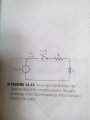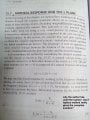# laplace transform method -type of solution

#### e-learner

Joined Apr 25, 2015
30
I know that laplace method provides a complete response ( for t≥0) circuit problems. Solution by this method provides exponential decaying terms also. Do exponential decaying terms 'not' represent the natural response?
I was reading another chapter of my book,( circuit analysis in s-domain), it says that forced reponse can be found using inverse laplace transform of the reponse function written in s-domain and the natural response is found by making use of poles of the that s-domain reponse function.

#### WBahn

Joined Mar 31, 2012
29,153
The natural response is simply the response of the system to its initial conditions in the absence of any forcing function. It may or may not have exponentially decaying terms. For instance, the natural response of an LC circuit (no resistance) oscillates, the natural response of a purely resistive circuit is simply zero (assuming you are looking for the voltage across or current through one of the resistors), and the natural response of an ideal inductor connected back on itself is a constant current with no decay.

•e-learner

#### e-learner

Joined Apr 25, 2015
30
The natural response is simply the response of the system to its initial conditions in the absence of any forcing function. It may or may not have exponentially decaying terms. For instance, the natural response of an LC circuit (no resistance) oscillates, the natural response of a purely resistive circuit is simply zero (assuming you are looking for the voltage across or current through one of the resistors), and the natural response of an ideal inductor connected back on itself is a constant current with no decay.
Should I imply that laplace provides solution for t≥0 and initial conditions at t=0- are not included, therfore I need to find the natural reponse using poles method?
Also, if initially a forcing function was present and then removed , does this means that should be no natural reponse in the system?

#### WBahn

Joined Mar 31, 2012
29,153
The forcing function establishes a set of conditions that apply at the moment the forcing function is removed. From that point on it responds naturally to those conditions.

•e-learner

#### e-learner

Joined Apr 25, 2015
30
one more question, when I solve from time domain to s-domain and then back to time domain, I get only the forced response and not the natural repsonse?

#### WBahn

Joined Mar 31, 2012
29,153
one more question, when I solve from time domain to s-domain and then back to time domain, I get only the forced response and not the natural repsonse?
What makes you think that? Particularly considering that the very first sentence of the first post says that you get the total response.

#### MrAl

Joined Jun 17, 2014
10,596
one more question, when I solve from time domain to s-domain and then back to time domain, I get only the forced response and not the natural repsonse?
Hi,

Can you show an example of the problem you are having?
The transform works with forcing functions as well, even very complex ones although we usually only need the simpler ones like the unit step and ramp for example.

The transform, for example, for the unit step is very simple:
u(t)=>1/s

Cant get any simpler than that. Then to apply this to your circuit, you must "convolve" that forcing function with your transfer function. Part of the theory is that a convolution in the time domain is equivalent to a multiplication in the frequency domain, so in this form you might have a transfer function like this:
Hs=1/(s+1)

and to convolve this with the unit step 1/s, we simply multiply:
Hout=(1/s)*(1/(s+1))

and the result looks like:
1/(s*(s+1))

Now when we take the inverse transform we get the time domain solution:
Vout(t)=1-e^(-t)

and that happens to be a low pass filter with a step input.

#### e-learner

Joined Apr 25, 2015
30this is the text that is leading me confusion.
There is an example on the next page (not clicked) in which there is no forcing function but initial conditions are known and current is to be found. I think pole method can be used in those cases when the forcing function is not known and you need to find the natural response.

#### WBahn

Joined Mar 31, 2012
29,153
There is a big difference between "no forcing function" and "forcing function is not known".